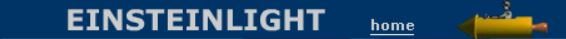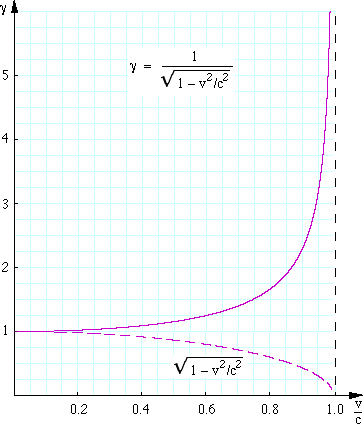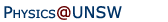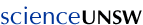Relativity in brief... or in detail..

# Time dilation and length contraction in Special Relativity

Time dilation, length contraction and the relativity of simultaneity are among the strange conclusions of special relativity. This page uses animations to explain them in more detail. There is a little mathematics: we use Pythagoras' theorem about the sides of a right angled triangle, but nothing beyond that.

• Time dilation,
• Pythagoras meets Einstein,
• Is time dilation true? How big are the effects?,
• Simultaneity,
• The limits to time order reversals,
• Length contraction and
• From special relativity to general relativity.

 Your browser does not support the video tag.

### Time dilation

In the animations below, Zoe is travelling at speed v as she drives past Jasper's verandah. She has various clocks on board: clocks that work using the laws of mechanics and/or electromagnetism. According to Einstein's principle of special relativity, these laws by which these clocks operate are the same for her and for Jasper, so it doesn't matter what sort of clock we choose. So let's choose a very simple electromagnetic clock. It uses electromagnetic waves and mirrors: it has a pulse of light going from side to side across her car, whose width is w. Zoe has timing apparatus to measure the time between reflections in the windows. So does Jasper. (To be practical, the mirrors could be only partially reflecting so that some of the light gets out.)

Prior to the experiment, Zoe parks her car (a 1962 Holden, unexpectedly capable of relativistic speeds) next to Jasper's verandah so we can observe her clock, shown in the diagram at right. Coincidentally, the car and the verandah are the same length.

• The animation below shows Jasper's and Zoe's observations of the events. In Jasper's frame, Zoe is travelling left to right at v = 0.8*c, and the pulse of light traces the lines shown, each of which is the hypotenuse of a right angled triangle. In Zoe's frame, Jasper and his verandah are travelling right to left at speed v = 0.8*c, and the light pulse of the clock just goes sideways, to and fro across the car. The red counter next to Zoe shows how many clock ticks she reports; that next to Jasper counts the ticks he observes. For both observers, each window reflection is a tick of Zoe's clock. (You can use the 'step' function to check that this happens. You can also check the constancy of the speed of light for the two observers by checking that the light pulse travels the same distance per frame.)

Now the speed of light c in each of the animations is the same - that's what the principle of relativity is all about. But the distances are different: for Jasper, each tick of Zoe's clock is the time T taken for light to trace the hypotenuse of the right angled triangle. For Zoe, the light pulse covers only w, the width of the car, in each tick. So the constant speed of light means that the light beam clock ticks at different rates for Jasper and for Zoe! From his frame, Jasper observes Zoe's clock running more slowly than Zoe does. (Remember, the red counters give the number of ticks of the clock as measured by each of the observers.) Let's call T' the time that Zoe measures for one tick of the clock, so

w = cT'.

### Pythagoras meets Einstein

How to relate, T, the time of a tick on Zoe's clock as Jasper observes it, to T', the time of a tick on the same clock, as Zoe sees it? Look at the triangle at the right of the animation above. Light travels at c, so the hypotenuse has length cT. One side of the triangle is the width w of the car. The other side is vT, where v is the speed of the car relative to Jasper. Pythagoras' theorem says that the square of the hyptenuse is the sum of the squares on the other two sides. Here, this gives
(cT)2 = w2 + (vT)2.Combining the two equations above: (cT)2 = (cT')2 + (vT)2,     then dividing by c2 and rearranging gives T' 2 = T2 - (v/c)2T2    whence T' = T(1 - (v/c)2)1/2 = T/γ,    where γ = 1/(1 - (v/c)2)1/2. So Jasper observes Zoe's clock to tick more slowly by a factor γ, which is always greater than or equal to one. This factor γ occurs regularly in special relativity, so we have plotted it at right. (The dashed line plots 1/γ.) We notice that, unless v is a substantial fraction of c, γ is approximately 1. This of course is why we don't notice time dilation at ordinary speeds. For an airplane travelling near the speed of sound, γ = 1.0000000000005. Note that 1/γ(v/c) is the equation of a circle, although we have stretched the horizontal axis so that the dashed line looks like an elipse. So γ (the solid line) is conveniently remembered as the reciprocal of a circle. In the animations, Zoe's car travels at 0.8c, so γ = 1.67, so Jasper measures Zoe's clock to have ticks that are 67% longer than the time that Zoe measures. What about other clocks? The choice of this rather peculiar clock was made only because it is one that so clearly depends on a simple electromagnetic phenomena. Other clocks (quartz crystals, springs, even Zoe's biological clock) depend on complicated combinations of electromagnetic phenomena such as the forces between atoms and molecules, and on Newton's laws. If they didn't differ from Jasper's clocks by the same factor of γ, then we would conclude that the laws of mechanics and/or electromagnetism are different between the two frames, contrary to the principle of relativity. So yes, time dilation would affect biological clocks as well, and Jasper thinks that Zoe is getting older more slowly than he is. Symmetry. But Zoe also thinks that Jasper is getting older more slowly than she is. (The situation between Jasper and Zoe is symmetrical: if you believe that a '62 Holden can travel at relativistic speeds, you might as well believe that the verandah can, too.) So Zoe observes that Jasper's clocks are running slow by γ, too. Hold on, I hear you say, how can both clocks run slow? Surely that's impossible? Puzzling, but not impossible, because the clock rates are measured in different frames. Let's suppose that they synchronise clocks when they pass each other. What happens when they compare time later? Now remember that their relative speed is a substantial fraction of the speed of light. Consequently, when Zoe looks at Jasper's clock, the light will take a while to reach her eyes. So she will see the time that the clock read when the light that she is seeing reflected off Jasper's clock. (Think of astronomers looking at very distant galaxies: they emitted the light we see when they and the universe was much younger than it is now.) So distant observes can't just use the time that they see on the clock, they have to correct this for the time of transmission of light (or radio etc) that is used to send the local time. This synchronisation effect is important enough to need another page on its own, because it is tempting to try to use this symmetry to create a paradox that would disprove relativity. See the twin paradox for an explanation. We call the time measured by an observer in his/her own frame the proper time. ('Proper' here means 'belonging to' or 'property of', it doesn't mean 'correct'.) So the time measured by other observers (Jasper in this example) is γ times greater than the proper time (Zoe's time in this example). γ is always greater than or equal to one.

### Is time dilation true? How big are the effects?

Do clocks at speed really run slower, and do people or things travelling at speed live longer?

One question at a time. Yes, clocks do run more slowly. Planes travel about a million times more slowly than c (so γ is about 1.0000000000005), but atomic clocks are very precise and so this tiny effect effect can actually be measured. In 1971, J. Haefele and R. Keating took atomic clocks on airliners travelling both East (with the Earth rotating underneath them in the direction of their motion over the Earth) and West (these planes have the Earth's rotation speed opposite that of their terrestrial motion, so the two tend to cancel). Apart from some complications due to the gravitational field variations and their acceleration (which are dealt with by general relativity), this is like the twin paradox, and it gave results in agreement with the relativistic prediction. (See the original paper by J.C. Hafele and R. E. Keating, Science 177, 166 (1972) for details. Also see the diagrams and discussion about this experiment and its complications on the FAQ in high school physics.)

Do people age more slowly? We don't know whether people age more slowly, because even cosmonauts don't travel fast enough for the effect to be statistically observable on their life spans*. However, people's ages are determined by physical and chemical processes in our bodies. Certainly we expect that people would age more slowly at relativistic speeds. Particles certainly do. Particle accelerators generate some short lived particles (eg muons or pions) that travel within a fraction of a percent of c, and (in the laboratory frame) they survive for much longer than their lifetime when at rest in the lab frame. Muons with a half life of 1.5 microseconds are also created several tens of km above the Earth in the upper atmosphere by cosmic rays. Travelling 50 km at c would take 170 microseconds or 110 half lives, so we should expect their numbers to be reduced by a factor of 2110 ~ 1033 (ie effectively none) to reach the surface. In fact they are measured at sea level and at various altitudes, with rates that agree with the relativistic dilation of their half lives. Time dilation happens, however counter-intuitive it may seem at first.

* Low orbits are the fastest, travelling around the Earth in about 90 minutes, which gives γ of about 1.0000000003. Suppose that a cosmonaut spent 2 years in space. Time dilation due to special relativity (neglecting general relativistic effects) would give an expected lifetime increase of 20 milliseconds. Lives, let alone life expectancies, are not measured that precisely!

How big are time dilation effects? Note the shape of the curve above: γ only starts to become large at speeds close to c. At 0.99*c, γ is 7. But in many modern devices, electrons are accelerated to higher speeds than this. In a typical electron accelerator used to treat cancers, the electrons have an energy of 20 MeV (see Module 5). The speed of such electrons is 0.9997*c and γ is 40.

Now of course an electron cannot go much faster than this, but it can have a lot more energy. In the Large Electron-Positron collider in Europe's nuclear research lab CERN, electrons (and positrons, or anti-electrons) were accelerated to energies of 100 GeV. For such particles, v = 0.999 999 999 95*c and γ is 200,000. Yes, time is slowed down by that factor. And the momentum is increased by that factor too: something that is rather important in the design of the collider because these electrons must be turned to go in a circle.

Nature can produce even larger particle energies. Some particles striking the Earth's upper atmosphere have energies that exceed 2*1020 eV. If such particles are protons (with mass of about 1 GeV), their speeds would be 0.999 999 999 999 999 999 999 995 c. For them, γ is 1011. Now the age of the universe is about 13 billion years for us, but for such particles, the age of the universe would be about (13 billion  years/1011), ie about a month. Such a particle could cross the visible universe in a matter of months (their time).

### From special relativity to general relativity

This presentation concerns only special relativity, not general relativity. Nevertheless,this is a good place to recommend a lovely paper by Sam Drake: The equivalence principle as a stepping stone from special relativity to general relativity: A Socratic dialog.

### Simultaneity

Having clocks that run at different rates leads to other strange effects: simultaneity is relative. Whether or not two things are simultaneous depends upon your frame of reference. The time order of events that are close together in time but distant in space can be different in different frames. You have probably noticed this in the preceding animation: in general, Jasper and Zoe do not agree on the time at which the light pulse is reflected.

In the next animation, Jasper has set up an apparatus to make simultaneous 'events'. A little while after he presses the switch, sparks jump at the two gaps, and two pulses of light travel towards him. (Note that the sparks are not simultaneous with the switch: the electric field in the circuit cannot travel faster than light.) The spark gaps are equidistant from Jasper, the light pulses arrive simultaneously, so he concludes that the two events (the two spark emissions) occurred simultaneously. As we'll see later, relative simultaneity can only be noticeable for events that are well separated in space, but close in time. For this reason, we asked Jasper to set up the spark gaps a long way apart, and so as to observe the small time effect, we've slowed everything down in this animation, compared to the previous ones, just for clarity.

Zoe also receives the two pulses of light, which she observes to come towards her at the same* speed c. Jasper has timed the pushing of the switch so that the two pulses, Zoe and Jasper all meet at the centre of the verandah at the same time*. But Zoe sees the light pulses emitted from Jasper's moving verandah, and in her frame of reference the two events (the two flashes of light) are not equidistant. For the two pulses to arrive simultaneously, Zoe deduces that the right hand pulse (emitted from the approaching spark gap) must have been emitted before the emission of the left hand pulse (the receding one), because of their relative motion.

* Why have we added this extra symmetry? When different observers compare results, it is very convenient if they can measure time from the moment when they meet because, as we shall see, simultaneity only has an absolute meaning for events that are not separated in space. So the usual convention in relativity is to measure time and space with respect to a single event. If you'd like to examine a less symmetric comparison, see Question 9 on the quiz.

Another non-intuitive result: events that are simultaneous to one observer need not be simulatenous to another. Indeed, the time order may be reversed: a traveller going from right to left with respect to Jasper would, by symmetry, observe the left hand pulse to be emitted first. (A question for you to puzzle on: look at when the switch near Jasper closes and work out why the sparks occur simultaneously for Jasper but not for Zoe. Answer below.)

* How can they seem to travel at c with respect to Jasper and also at c with respect to Zoe? Shouldn't Zoe see the light travelling at c-v or c+v? No it doesn't: all observers obesrve the light to travel at c. Yes, it's weird, but that's Einstein's principle of relativity: the laws of physics, including Maxwell's laws of electromagnetism, are the same for two observers in inertial frames. (For a derivation of the expressions for relative velocities see Lorentz transforms, the addition of velocities and spacetime.)

Sometimes one encounters this objection: in this example, Jasper, sends a pulse of voltage down wires to create his two simultaneous events. What if, instead, he switched two distant switches using two very long, rigid rods. Wouldn't they be simultaneous then? The key word here is "rigid". When you push on the end of a rod of length L, the other end does not move instantaneously. It takes a time L/v, where v is either the speed of sound in the object or the speed of a shock wave in the object. The speed of sound in solids is typically a few km/s. (It is the square root of the ratio of an elastic modulus to the density.) Interatomic forces are continuous functions of separation (see Young's modulus, Hooke's law and material properties), you cannot make an infinitely rigid rod, i.e. you cannot make one with an infinite elastic modulus and thus an infinite v. So, although Jasper might see a mechanical wave travel along each rod at the same speed, Zoe would point out that, to her, the waves have different speeds.

### The limits to time order reversals

But isn't the relativity of simultaneity and time order impossible? Does it mean that some observer can determine that my death happened before I was born?

No. As we show elsewhere, if two events are separated by distance L (according to one observer) and time difference Δt (according to the same observer), then their time order can only be reversed for some other observer if L is greater than cΔt. Let's suppose that a cosmonaut dies 80 years after his birth (Δt = 80 years). For an observer to deduce that he died before he was born, his birth and death would have to be separated in space by more than cΔt, which is 80 light years. But (assuming the cosmonaut is present at both his birth and death — even the busiest people manage to attend both!) to get from the place of his birth to the place of his death, he would have to travel more than 80 light years (L greater than 80 light years) in 80 years. He would have to travel at L/Δt, which is greater than the speed of light, which is in turn impossible. (For quantitative details, see Lorentz transforms, the addition of velocities and spacetime.)

### Length contraction

You have probably noticed that, in Jasper's version of events, Zoe's car has shrunk. And vice versa. We haven't proved that yet, but it's logically simple. Suppose that Zoe and Jasper choose to measure lengths in lightyears, lightseconds, lightnanoseconds* etc: ie they measure distance by how long light takes to cover the distance. If they agree on the speed of light, but disagree on measurements of time, they must inevitably disagree on length as well. If you observe someone's clocks run slowly by a factor γ, you will also observe her rulers to be short by a factor of γ: that's the only way that she can measure the speed of light to have the same value you get.

* The lightnanosecond is a convenient unit. c is about 3 108 metres per second, and a nanosecond is 10-9 seconds, so a lightnanosecond is 0.3 metres. (Americans, who use British imperial units, can therefore remember that the speed of light is about one foot per nanosecond. The rest of us can remember it as 30 centimetres per nanosecond.)
Zoe, who is a graffiti artist in her spare time, will demonstrate this: she decides to tag the two ends of the verandah. (The paint can is green, and it sprays purple paint.) For Jasper, the distance between the tags will be his proper length, ie the length measured in his frame, because they are stationary with respect to him. Zoe can measure the time between the two tags, and thus get her measurement of the length of the verandah.

Both agree that the time between the two tags — the time Zoe takes to go past the verandah — is two ticks of Zoe's clock. This is 2T' for Zoe, so the length that Zoe measures is 2vT'. But for Jasper, two ticks of Zoe's clock takes 2T = 2T'γ. The length that Jasper measures for the verandah is 2vT = 2vT'γ. Jasper measures the verandah to be γ times longer than Zoe measures it.

Further, the situation is symmetrical: Jasper observes the car to be shrunk with respect to the verandah, while Zoe concludes that the verandah has shrunk with respect to the car. The proper length is always longer than a measure of the length from another frame. But can't one make a paradox from this? See the "pole in the barn" paradox.

Severe simplifications have been made in the animations shown above. Even if cars could travel at relativistic speeds, this is not how they would "look", because of aberrations associated with the finite time of flight. See References and caveats for more information.

Answer to puzzle. Variations in the electric field in the circuit travels at approximately the speed of light, so the step change in voltage (ΔV) caused by closing the switch travels at this speed to the spark gaps. (For simplicity, we neglected the length of the common part of the circuit — the vertical lines on the diagram — when scaling the animation, because this could be made very short.) For Jasper, this ΔV is travelling (at c) towards two stationary spark gaps. For Zoe, it is travelling (at c) towards two spark gaps moving at 0.8*c. So for Zoe, ΔV arrives at the right hand spark gap first. A caution: although electromagnetic fields, including ΔV, in and around an electric circuit may travel at speeds near that of light, this is not true of electrons. Electron speed in most circuits is only a tiny fraction of c. Exceptions are the electron beams in high energy accelerators.

 FAQ. How is the 1961 EK Holden capable of relativistic speeds? This has never been satisfactorily explained. Zoe's car has neither speed stripes nor spoiler, so that can't be the answer. However, as the fins on this model serve no other purpose, we speculate that they may be involved. Late news. Reader Kevin Jezorek writes: "I have been reading your article on time dilation, and I've noticed an inconsistency in a particular detail of the example. In the introduction, Zoe is claimed to be operating a 1962 Holden. The footnotes describe a 1961 Holden which is not capable of relativistic speeds. This is correct, the relativistic speed propulsion unit was not available on the Holden until 1962. I have a feeling Zoe was driving a '62 Holden the entire time."Home | Summary | Quiz | Credits School of Physics - The University of New South Wales Sydney, Australia 2052 © School of Physics UNSW 2052 Disclaimer feedback